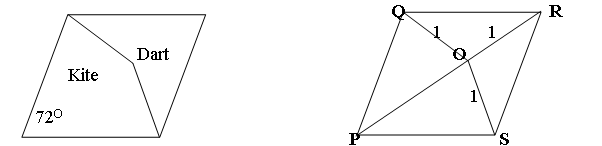#### You may also like### Golden Thoughts

Rectangle PQRS has X and Y on the edges. Triangles PQY, YRX and XSP have equal areas. Prove X and Y divide the sides of PQRS in the golden ratio.### Pent

The diagram shows a regular pentagon with sides of unit length. Find all the angles in the diagram. Prove that the quadrilateral shown in red is a rhombus.### Pentakite

Given a regular pentagon, can you find the distance between two non-adjacent vertices?

# Darts and Kites

##### Age 14 to 16Challenge LevelThe diagram shows a rhombus $PQRS$ with an internal point $O$ such that $OQ = OR = OS = 1$ unit. Penrose used this rhombus, split into two quadrilaterals, a dart and a kite, to make his famous tiling which fills the plane but, unlike a tessellation, does not repeat itself by translation or rotation.

Find all the angles in the diagram, show that $POR$ is a straight line and show that triangles $PRS$ and $QRO$ are similar. Hence prove that the length of the side of the rhombus is equal to the Golden Ratio $(1+ \sqrt{5})/2$.